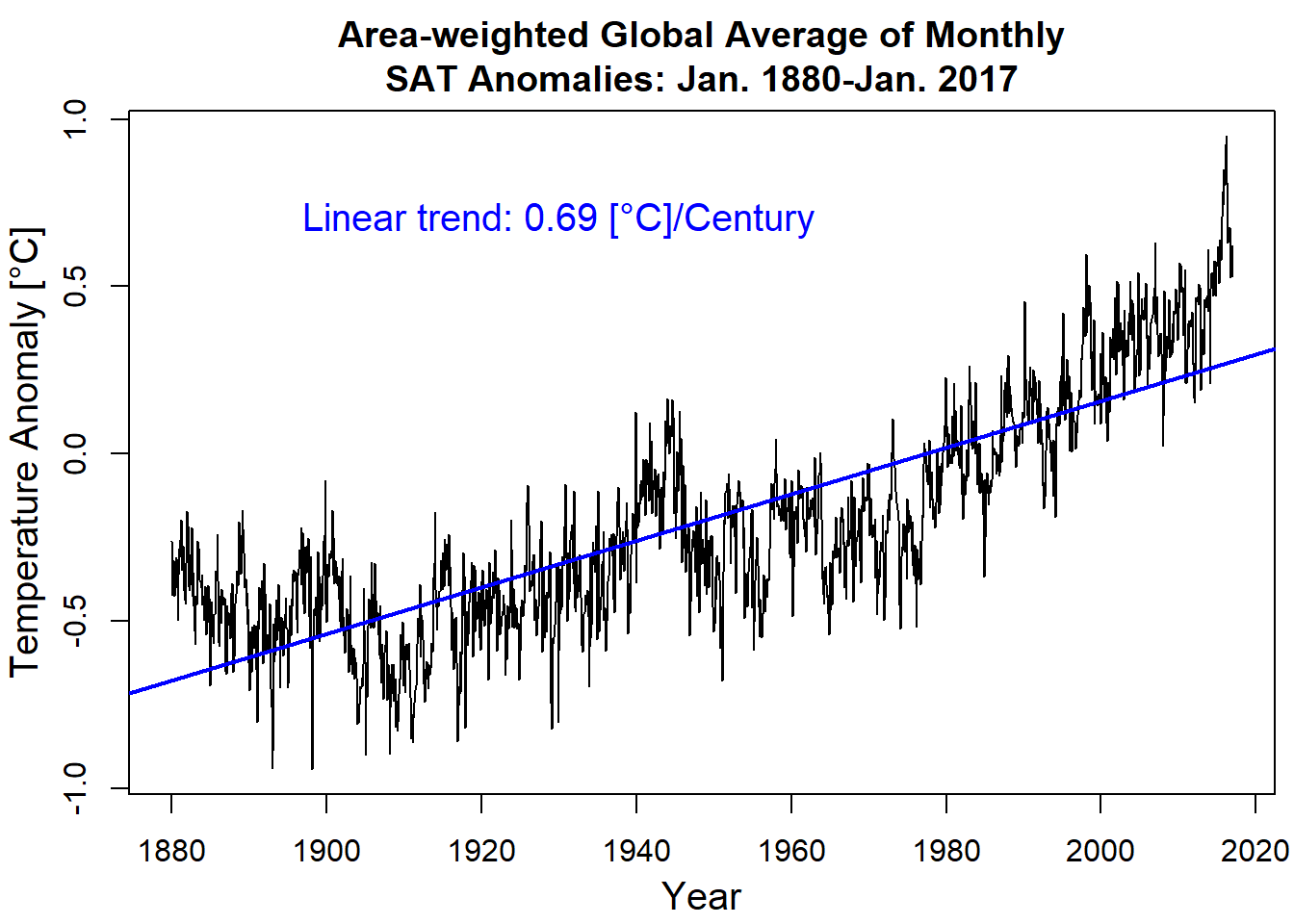# Theory and Applications

### SSP Shen and RCJ Somerville

Version 1.0 released in July 2019 and coded by Dr. Samuel Shen, Distinguished Professor
San Diego State University, California, USA
Email:

Version 2.0 compiled and updated by Momtaza Sayd
San Diego State University May 2021
Version 3.0 compiled and updated by Joaquin Stawsky
San Diego State University June 2022

## Chapter 11: Climate Data Matrices and Linear Algebra

### Read NOAAGlobalTemp and form the space-time data matrix

``````#NOAAGlobalTemp dataset since 1880: A merged land SAT and ocean SST anomalies
#The monthly 5-deg gridded data and their global average can be
#The gridded data has 43MB
#The data can also be downloaded from the website of this book
#https://climatemathematics.sdsu.edu
#The file name is NOAAGlobalTemp.gridded.v4.0.1.201701.asc

rm(list = ls(all = TRUE))
#.asc is an ASCII data format
#This book uses "scan" to read the asc data
#and then write the data into a space-time matrix
#Climate Mathematics treats space-time matrix as
#its data standard while big climate data use .nc

setwd("~/sshen/climmath/data")
da1 <- scan("NOAAGlobalTemp.gridded.v4.0.1.201701.asc")
#From Jan 1880 to Jan 2017
length(da1)``````
``##  4267130``
``````# 4267130
da1[1:3]``````
``##     1.0 1880.0 -999.9``
``````#    1.0 1880.0 -999.9 #means month, year, temp
#data in 72 rows (2.5, ..., 357.5) and
#data in 36 columns (-87.5, ..., 87.5)
tm1 <- seq(1,4267129, by = 2594)
tm2 <- seq(2,4267130, by = 2594)
length(tm1)``````
``##  1645``
``length(tm2)``
``##  1645``
``````mm1 <- da1[tm1] #Extract months
yy1 <- da1[tm2] #Extract years
``##  1 2 3 4 5 6``
``head(yy1)``
``##  1880 1880 1880 1880 1880 1880``
``length(mm1)``
``##  1645``
``length(yy1)``
``##  1645``
``````rw1 <- paste(yy1, sep = "-", mm1) #Combine YYYY with MM
``##      1  2595  5189  7783 10377 12971``
``head(tm2)``
``##      2  2596  5190  7784 10378 12972``
``````tm3 <- cbind(tm1,tm2)
tm4 <- as.vector(t(tm3))
``##     1    2 2595 2596 5189 5190``
``````#    1    2 2595 2596 5189 5190
da2 <- da1[-tm4] #Remote the months and years data from the scanned data
length(da2)/(36*72)``````
``##  1645``
``````# 1645 #months, 137 yrs 1 mon: Jan 1880-Jan 2017
da3 <- matrix(da2,ncol = 1645) #Generate the space-time data
#2592 ( = 36*72) rows and 1645 months ( = 137 yrs 1 mon)
dim(da3)``````
``##  2592 1645``
``````# 2592 1645

#Put space-time coordinates in the space-time data da3
colnames(da3) <- rw1
lat1 <- seq(-87.5, 87.5, length = 36)
lon1 <- seq(2.5, 357.5,  length = 72)
LAT <- rep(lat1, each = 72)
LON <- rep(lon1,36)
gpcpst <- cbind(LAT, LON, da3)
dim(gpcpst)``````
``##  2592 1647``
``````# 2592 1647 #The first two columns are Lat and Lon
#-87.5 to 87.5 and then 2.5 to 375.5
#The first row for time is header, not counted as data.
gpcpst[1:3,1:6] #Part of the data``````
``````##        LAT  LON 1880-1 1880-2 1880-3 1880-4
## [1,] -87.5  2.5 -999.9 -999.9 -999.9 -999.9
## [2,] -87.5  7.5 -999.9 -999.9 -999.9 -999.9
## [3,] -87.5 12.5 -999.9 -999.9 -999.9 -999.9``````
``````#       LAT  LON 1880-1 1880-2 1880-3 1880-4
#[1,] -87.5  2.5 -999.9 -999.9 -999.9 -999.9
#[2,] -87.5  7.5 -999.9 -999.9 -999.9 -999.9
#[3,] -87.5 12.5 -999.9 -999.9 -999.9 -999.9

write.csv(gpcpst,file = "NOAAGlobalT.csv")
#Output the data as a csv file``````

### Plot Fig. 11.4: Dec 2015 global surface temperature anomalies map

``library(maps) # install maps package first if not done before``
``## Warning: package 'maps' was built under R version 4.1.3``
``````Lat <- seq(-87.5, 87.5, length = 36)
Lon <- seq(2.5, 357.5, length = 72)
mapmat <- matrix(gpcpst[,1634],nrow = 72)
#column 1634 corresponding to Dec 2015
#Convert the vector into a lon-lat matrix for R map plotting
mapmat <- pmax(pmin(mapmat,6),-6) #Put values between -6 and 6
#Matrix flipping is not needed since the data go from 2.5 to 375.5
#plot.new()
par(mar = c(4,5,3,0))
int <- seq(-6,6,length.out = 81)
rgb.palette <- colorRampPalette(c('black','blue', 'darkgreen','green',
'yellow','pink','red','maroon'),interpolate = 'spline')
filled.contour(Lon, Lat, mapmat, color.palette = rgb.palette, levels = int,
plot.title = title(main = "NOAAGlobalTemp Anomalies Dec. 2015 [°C]",
xlab = "Longitude",ylab = "Latitude", cex.lab = 1.5),
plot.axes = {axis(1, cex.axis = 1.25);
axis(2, cex.axis = 1.25);map('world2', add = TRUE);grid()},
key.title = title(main = "[°C]"),
key.axes = {axis(4, cex.axis = 1.25)})``````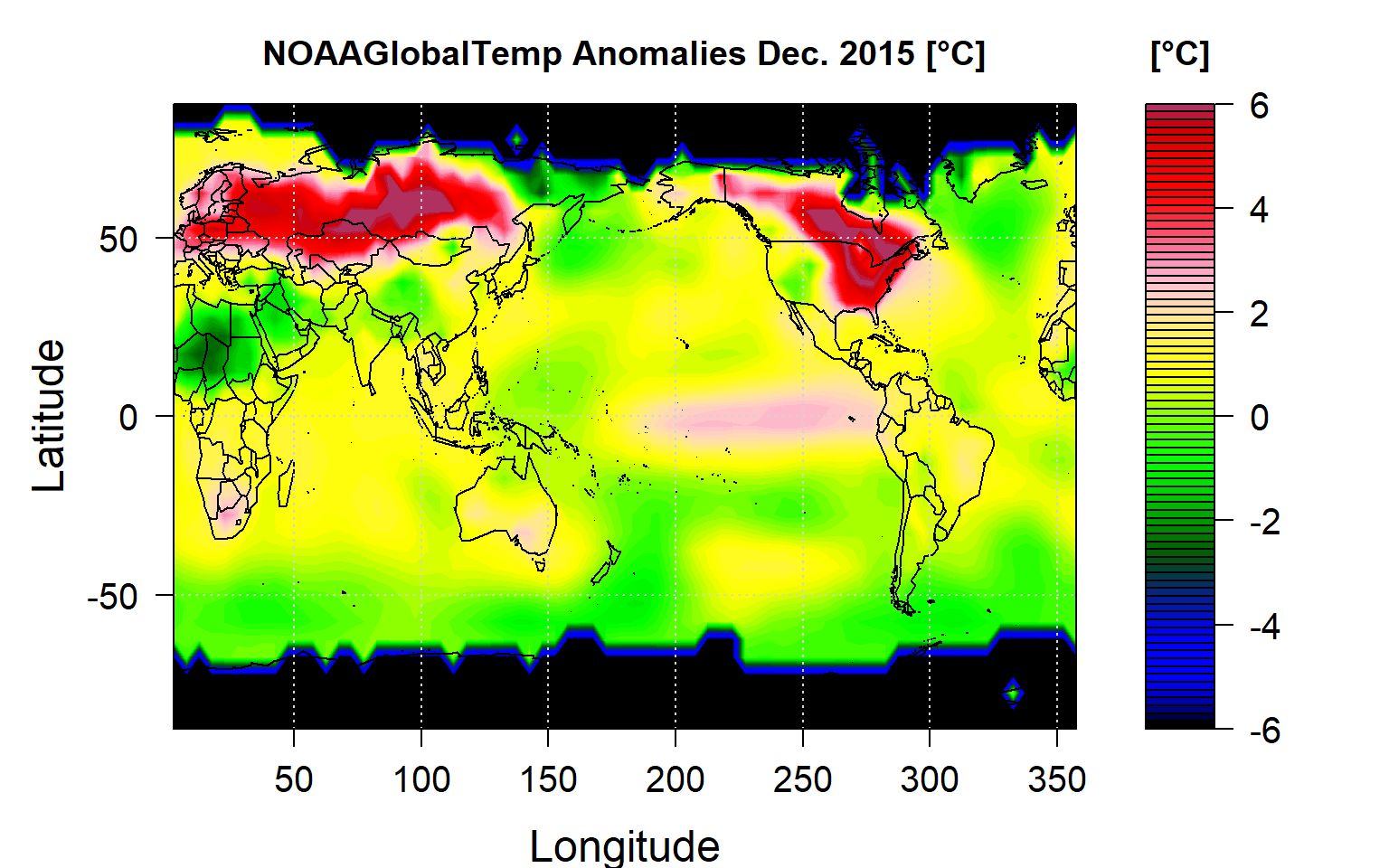### Plot Fig. 11.5: Dec 1997 tropical Pacific SST anomalies map

``````#Select only the data for the tropical Pacific region
n2 <- which(gpcpst[,1]>-20&gpcpst[,1]<20&gpcpst[,2]>160&gpcpst[,2]<260)
dim(gpcpst)``````
``##  2592 1647``
``length(n2)``
``##  160``
``````# 160 ( = 8 latitude bends and 20 longitude bends)
pacificdat <- gpcpst[n2,855:1454]

#plot.new()
Lat <- seq(-17.5,17.5, by = 5)
Lon <- seq(162.5, 257.5, by = 5)
par(mar = c(4,5,3,0))
mapmat <- matrix(pacificdat[,564], nrow = 20)
int <- seq(-5,5,length.out = 81)
rgb.palette <- colorRampPalette(c('black','blue', 'darkgreen',
'green', 'yellow','pink','red','maroon'),
interpolate = 'spline')
mapmat <- mapmat[,seq(length(mapmat[1,]),1)]
filled.contour(Lon, Lat, mapmat, color.palette = rgb.palette, levels = int,
xlim = c(120,300),ylim = c(-40,40),
plot.title = title(main = "Tropic Pacific SAT Anomalies [°C]: Dec. 1997",
xlab = "Longitude",ylab = "Latitude", cex.lab = 1.25),
plot.axes = {axis(1, cex.axis = 1.25); axis(2, cex.axis = 1.25);
key.title = title(main = "[°C]"),
key.axes = {axis(4, cex.axis = 1.25)})``````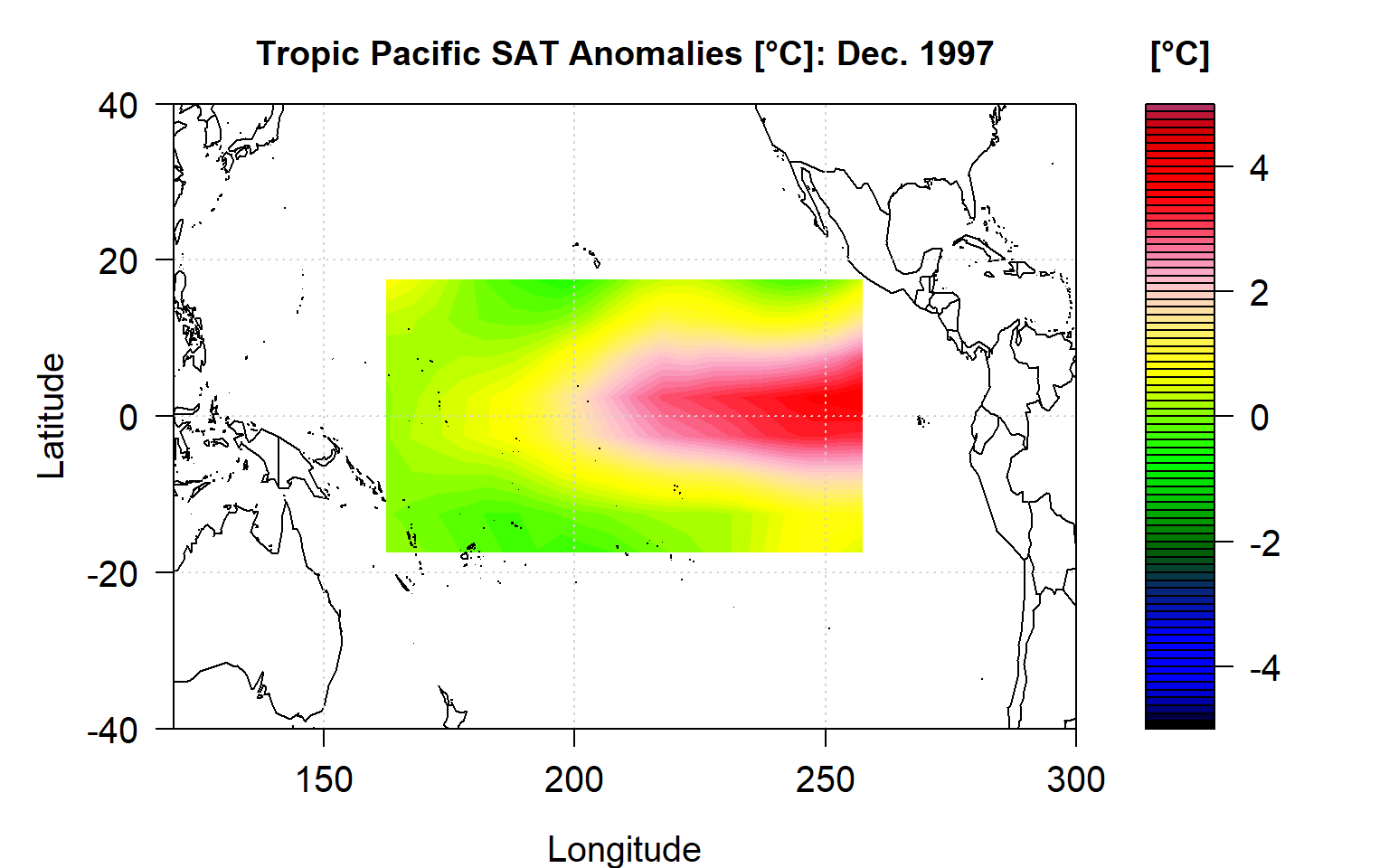``````#Extract data for a specified box with given lat and lon
n2 <- which(gpcpst[,1] == 32.5&gpcpst[,2] == 242.5)
SanDiegoData <- gpcpst[n2,855:1454]
plot(seq(1880,2017, len = length(SanDiegoData)),
SanDiegoData, type = "l",
xlab = "Year", ylab = "Temperature [°C]",
main = "San Diego Temperature Anomalies History")``````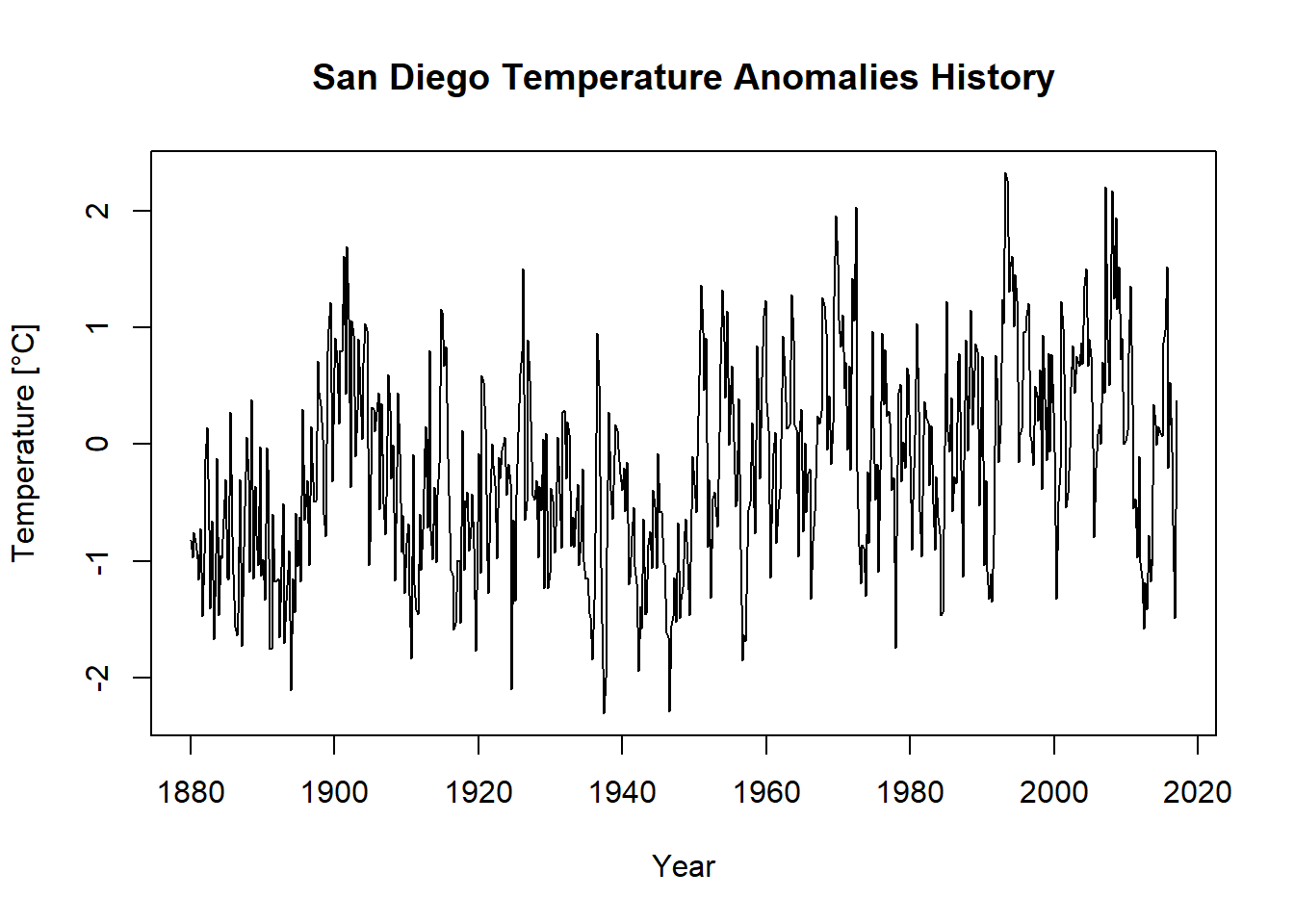``lm(SanDiegoData ~ seq(1880,2017, len = length(SanDiegoData)))``
``````##
## Call:
## lm(formula = SanDiegoData ~ seq(1880, 2017, len = length(SanDiegoData)))
##
## Coefficients:
##                                 (Intercept)
##                                  -15.060295
## seq(1880, 2017, len = length(SanDiegoData))
##                                    0.007624``````
``````n2 <- which(gpcpst[,1] == 52.5&gpcpst[,2] == 247.5)
EdmontonData <- gpcpst[n2,855:1454]
plot(seq(1880,2017, len = length(EdmontonData)),
EdmontonData, type = "l",
xlab = "Year", ylab = "Temperature [°C]",
main = "Temperature Anomalies History of Edmonton, Canada")``````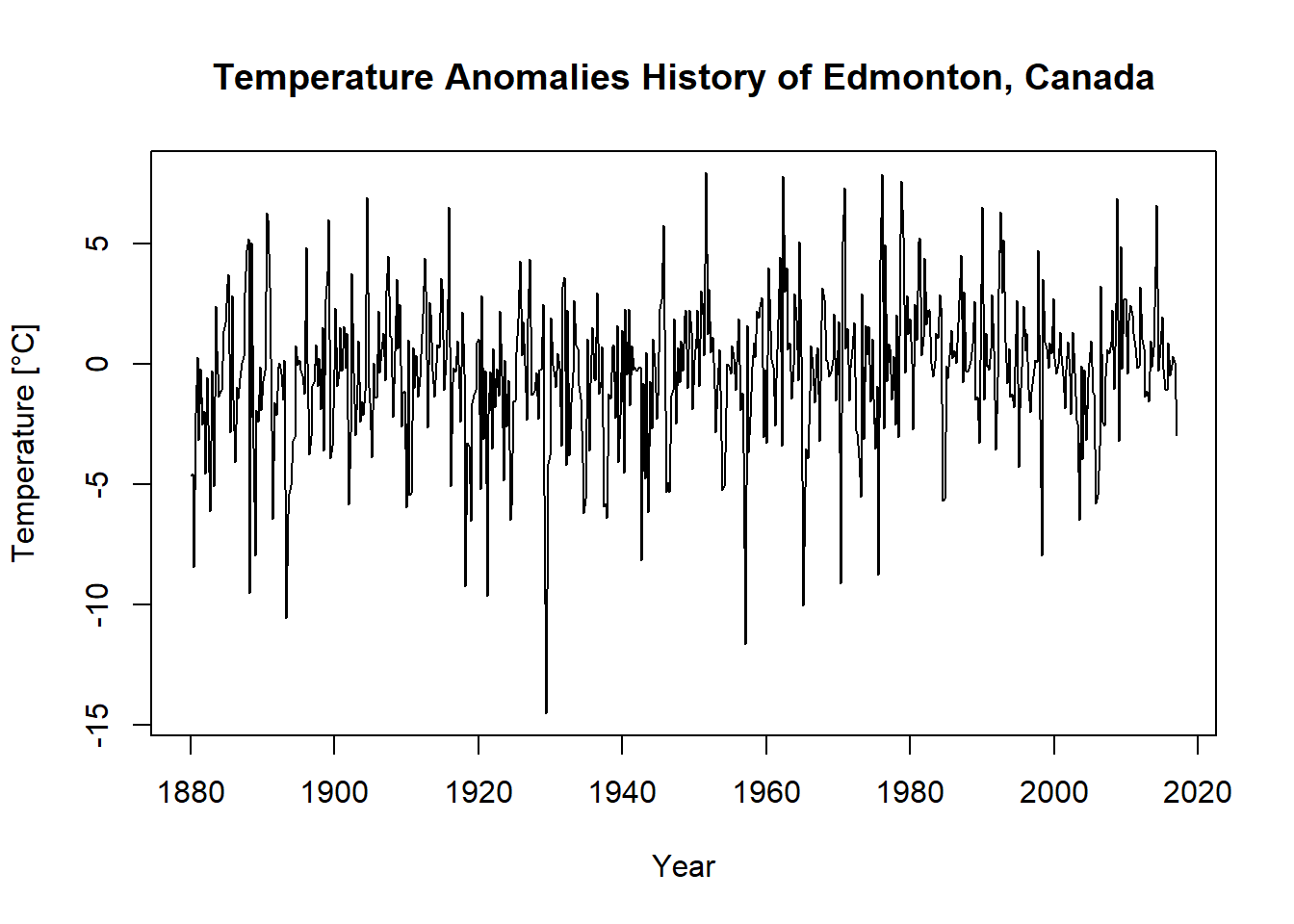``lm(EdmontonData ~ seq(1880,2017, len = length(EdmontonData)))``
``````##
## Call:
## lm(formula = EdmontonData ~ seq(1880, 2017, len = length(EdmontonData)))
##
## Coefficients:
##                                 (Intercept)
##                                   -23.26528
## seq(1880, 2017, len = length(EdmontonData))
##                                     0.01178``````
``````#Compute the area-weighted average of the gridded data
#36-by-72 boxes and Jan1880-Jan2016 = 1633 months + lat and lon
#Compute the area-weight for each box and each month
#that has data. Thus the area-weight is a matrix.
areaw <- matrix(0,nrow = 2592,ncol = 1647)
dim(areaw)``````
``##  2592 1647``
``````# 2592 1647
temp <- gpcpst
areaw[,1] <- temp[,1]
areaw[,2] <- temp[,2]
veca <- cos(areaw[,1]*pi/180) #convert deg into radian
#create an area-weight matrix equal to cosine for the box with data
#and zero for the box with missing data
for(j in 3:1647) {for (i in 1:2592) {if(temp[i,j]> -290.0) {areaw[i,j] = veca[i]} }}

#area-weight data matrix's first two columns as lat-lon
tempw <- areaw*temp
tempw[,1:2] <- temp[,1:2]
#create monthly global average vector for 1645 months
#Jan 1880-Jan 2017
avev <- colSums(tempw[,3:1647])/colSums(areaw[,3:1647])``````

### Plot Fig. 11.6: Global average temperature and its trend

``````plot.new()
timemo <- seq(1880,2017,length = 1645)
par(mar = c(3.5,3.5,3,0.5))
par(mgp = c(2.3,1.0,0.0))
plot(timemo,avev,type = "l", cex.lab = 1.25,
xlab = "Year", ylab = "Temperature Anomaly [°C]",
main = "Area-weighted Global Average of Monthly\nSAT Anomalies: Jan. 1880-Jan. 2017")
abline(lm(avev ~ timemo),col = "blue",lwd = 2)
text(1930,0.7, "Linear trend: 0.69 [°C]/Century",
cex = 1.25, col = "blue")``````